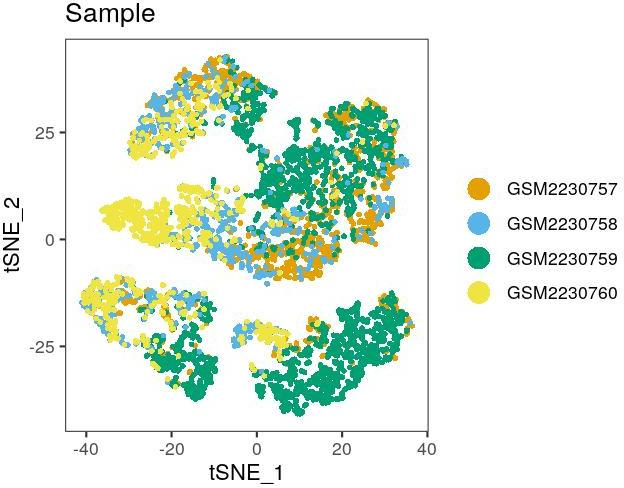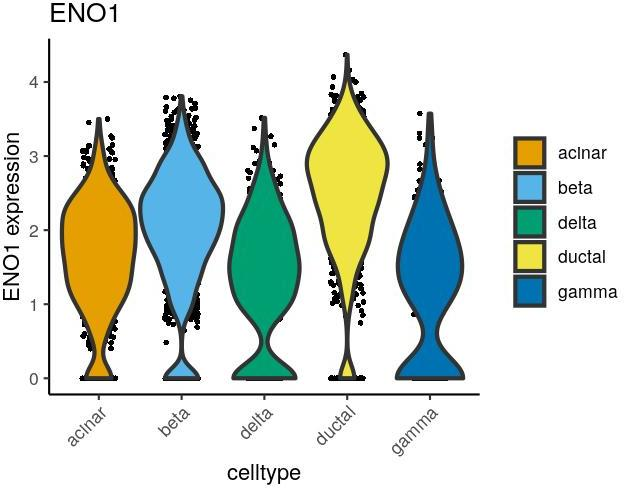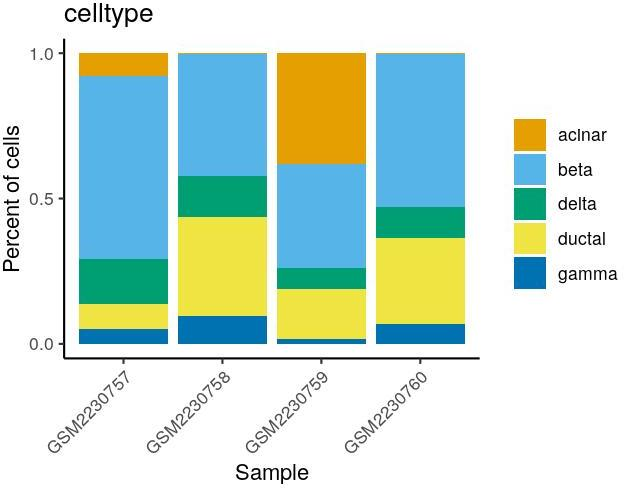# 1 Introduction

dittoSeq is a tool built to enable analysis and visualization of single-cell and bulk RNA-sequencing data by novice, experienced, and color-blind coders. Thus, it provides many useful visualizations, which all utilize red-green color-blindness optimized colors by default, and which allow sufficient customization, via discrete inputs, for out-of-the-box creation of publication-ready figures.

For single-cell data, dittoSeq works directly with data pre-processed in other popular packages (Seurat, scater, scran, …). For bulk RNAseq data, dittoSeq’s import functions will convert bulk RNAseq data of various different structures into a set structure that dittoSeq helper and visualization functions can work with. So ultimately, dittoSeq includes universal plotting and helper functions for working with (sc)RNAseq data processed and stored in these formats:

Single-Cell:

• Seurat (versions 2 & 3)
• SingleCellExperiment

Bulk:

• SummarizedExperiment (the general Bioconductor Seq-data storage system)
• DESeqDataSet (DESeq2 package output)
• DGEList (edgeR package output)

For bulk data, or if your data is currently not analyzed, or simply not in one of these structures, you can still pull it in to the SingleCellExperiment structure that dittoSeq works with using the `importDittoBulk` function.

## 1.1 Color-blindness friendliness:

The default colors of this package are red-green color-blindness friendly. To make it so, I used the suggested colors from (Wong 2011) and adapted them slightly by appending darker and lighter versions to create a 24 color vector. All plotting functions use these colors, stored in `dittoColors()`, by default.

• Shapes displayed in the legends are generally enlarged as this can be almost as helpful as the actual color choice for colorblind individuals.
• When sensible, dittoSeq functions have a shape.by input for having groups displayed through shapes rather than color. (But note: even as a red-green color impaired individual myself writing this vignette, I recommend using color and I generally only use shapes for showing additional groupings.)
• dittoDimPlots can be generated with letters overlaid (set do.letter = TRUE)
• The `Simulate` function allows a cone-typical individual to see what their dittoSeq plots might look like to a colorblind individual.

## 1.2 Disclaimer

Code used here for dataset processing and normalization should not be seen as a suggestion of the proper methods for performing such steps. dittoSeq is a visualization tool, and my focus while developing this vignette has been simply creating values required for providing visualization examples.

# 2 Installation

dittoSeq is available through Bioconductor.

``````# Install BiocManager if needed
if (!requireNamespace("BiocManager", quietly = TRUE))
install.packages("BiocManager")

# Install dittoSeq
BiocManager::install("dittoSeq")``````

## 2.1 Some setup for this vignette that can be ignored

Here, we will need to do some prep as the dataset we will use from Baron et al. (2016) is not normalized nor dimensionality reduced.

``````library(dittoSeq)
library(scRNAseq)
library(SingleCellExperiment)
library(Seurat)
sce <- BaronPancreasData()
# Trim to only 5 of the cell types for simplicity of vignette
sce <- sce[,meta("label",sce) %in% c(
"acinar", "beta", "gamma", "delta", "ductal")]``````

Now that we have a single-cell dataset loaded, we are ready to go. All functions work for either Seurat or SCE encapsulated single-cell data.

But to make full use of dittoSeq, we should really have this data log-normalized, and we should run dimensionality reduction and clustering.

``````# Make Seurat and grab metadata
seurat <- CreateSeuratObject(counts(sce))
PercentageFeatureSet(seurat, pattern = "^MT"),
col.name = "percent.mt")
# Basic Seurat workflow (possibly outdated, but fine for this vignette)
seurat <- NormalizeData(seurat, verbose = FALSE)
seurat <- FindVariableFeatures(object = seurat, verbose = FALSE)
seurat <- ScaleData(object = seurat, verbose = FALSE)
seurat <- RunPCA(object = seurat, verbose = FALSE)
seurat <- RunTSNE(object = seurat)
seurat <- FindNeighbors(object = seurat, verbose = FALSE)
seurat <- FindClusters(object = seurat, verbose = FALSE)``````
``````# Grab PCA, TSNE, clustering, log-norm data, and metadata to sce

# sce <- as.SingleCellExperiment(seurat)
# At the time this part of the vignette was made, the above function gave
# warnings. So... manual method
sce, embeddings = Embeddings(seurat, reduction = "pca"), name = "PCA")
sce, embeddings = Embeddings(seurat, reduction = "tsne"), name = "TSNE")
sce\$idents <- seurat\$seurat_clusters
assay(sce, "logcounts") <- GetAssayData(seurat)
sce\$percent.mt <- seurat\$percent.mt
sce\$celltype <- seurat\$celltype
sce\$Sample <- seurat\$Sample``````

Now that we have a single-cell dataset loaded and analyzed in Seurat, let’s convert it to an SCE for examples purposes.

All functions will work the same for either the Seurat or SCE version.

# 3 Getting started

## 3.1 Single-cell RNAseq data

dittoSeq works natively with Seurat and SingleCellExperiment objects. Nothing special is needed. Just load in your data if it isn’t already loaded, then go!

``dittoDimPlot(seurat, "Sample")````dittoPlot(seurat, "ENO1", group.by = "celltype")````dittoBarPlot(sce, "celltype", group.by = "Sample")``## 3.2 Bulk RNAseq data

dittoSeq works natively with bulk RNAseq data stored as a SummarizedExperiment object. For bulk data stored in other forms, namely as a DGEList or as raw matrices, one can use the `importDittoBulk()` function to convert it into the SingleCellExperiment structure.

Some brief details on this structure: The SingleCellEExperiment class is very similar to the SummarizedExperiment class, just with room added for storing pre-calculated dimensionality reductions.

``````# First, let's make some mock expression and conditions data
exp <- matrix(rpois(20000, 5), ncol=20)
colnames(exp) <- paste0("sample", seq_len(ncol(exp)))
rownames(exp) <- paste0("gene", seq_len(nrow(exp)))
logexp <- logexp <- log2(exp + 1)

conditions <- factor(rep(1:4, 5))
sex <- c(rep("M", 9), rep("F", 11))``````

### 3.2.1 Standard bulk data import workflow, `importDittoBulk()`

Importing bulk data can be accomplished with just the `importDittoBulk()` function. The function converts various common storage structures for bulk data into the `SingleCellExperiment` structure.

``````# Import
myRNA <- importDittoBulk(
# x can be a DGEList, a DESeqDataSet, a SummarizedExperiment,
#   or a list of data matrices
x = list(counts = exp,
logcounts = logexp),
# Optional inputs:
sex = sex),
reductions = list(pca = matrix(rnorm(20000), nrow=20)))``````

Metadata and dimensionality reductions can be added either directly within the `importDittoBulk()` function via the `metadata` and `reductions` inputs, respectively, or separately afterwards:

``````# Add metadata (metadata can alternatively be added in this way)
myRNA\$conditions <- conditions
myRNA\$sex <- sex

``dittoDimPlot(myRNA, "sex", size = 3, do.ellipse = TRUE)``